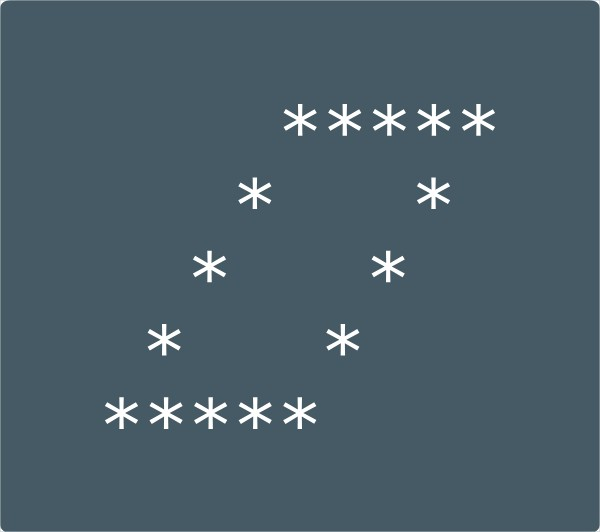# Hollow Rhombus Star Pattern Program In C | Patterns

Hollow Rhombus star pattern program in C – In this distinct article, we will explain how to print a hollow rhombus star pattern in C Programming.

A bunch of methods are used to print the Hollow Rhombus Star Pattern in C Programming such as:

• Using For Loop
• Using While Loop
• Using Do-While Loop

As we all know, Rhombus is a quadrilateral, which essentially means a 2-dimensional figure which is bound with four sides. The characteristics of a Rhombus are opposite sides of a rhombus are parallel to each other.

All the sides of a rhombus are equal in nature. The opposite angles of a rhombus are also equal in nature. Owing to these features, the diagonals of a rhombus also cut each other at right angles.

A Hollow Rhombus Star Pattern looks like this:In this piece, followers have to print a star pattern. Star patterns are basically the rudimentary level of teaching logic and how to use it in the world of programming.

Also, check out the sample outputs, sample programs below.

## Using For Loop

1) Read the number of rows and store that value into the variable n, read the entered symbol using getchar() function and store it in the variable ch.

2) The outer for loop iterates through rows from i=1 to n with the structure for(i=1;i<=n;i++).

3) The 1st inner for loop iterates through columns from j=1 to n-i with the structure for(j=1;j<=n-i;j++) and prints space.

4) The 2nd inner loop iterates through columns from j=1 to n with the structure for(j=1;j<=n;j++) and prints symbol for i=1 or n.

5) For i!=1 or i!=n, the 3rd inner loop iterates through columns from j=1 to n.

a) Prints symbol if j=1 or n.b) Otherwise it print space.

6) Cursor comes to next line for each iteration of i.

Output:

### Using While Loop

1) The outer while loop iterates through rows until the condition i<=n becomes false.

2) The 1st inner while loop iterates through columns and prints space until the condition j++<=n-i becomes false.

3) For i=1 or n the 2nd inner while loop iterates through columns and prints symbol until the condition j<=n becomes false.

4) At remaining rows other than 1,n.The 3rd inner while loop iterates through columns until the condition j<=n becomes false.

a) Prints symbol if j=1 or n.b) Otherwise prints space.

5)Cursor comes to the next line for each iteration of the outer while loop.

Output:

#### Using Do-While Loop

1) For i=1 the outer do-while loop iterates one time.

2) a) For j=1 the 1st inner do-while loop prints one space.

b) j value increased by 1.

c) Checks the condition j++<=n-i.If the condition is true then loop iterates through column again. Repeats until the condition becomes false.

3) If i=1 or n(1st row or nth row)

a) The 2nd inner do-while loop prints one symbol for j=1.

b) j value increased by 1.

c) Checks the condition ++j<=n.If the condition is true then loop iterates once through the column.Repeats until the condition becomes false.

4) If i!=1 or i!=n (other than the 1st row, last row)

a) The 3rd inner do-while loop prints one character if j=1 or n.Otherwise, it prints space.

b) j value increased by 1.

c) Checks the condition ++j<=n.If the condition is true loop iterates once.Repeats until the condition becomes false.

5) Cursor comes to the next line for each iteration.

6) a) i value increased by 1.

b) Checks the condition i<=n.If the condition true loop iterates once.Repeats until the condition becomes false.

Output:
x

## C Program To Print Number Of Days In A Month | Java Tutoring

C program to input the month number and print the number of days in that ...